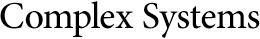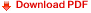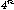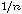## Scaling Relationships in Back-propagation LearningGerald Tesauro
Bob Janssens
Center for Complex Systems Research, University of Illinois at Urbana-Champaign
508 South Sixth Street, Champaign, IL 61820, USA

#### Abstract

We present an empirical study of the required training time for neural networks to learn to compute the parity function using the back-propagation learning algorithm, as a function of the number of inputs. The parity function is a Boolean predicate whose order is equal to the number of inputs. We find that the training time behaves roughly aswhereis the number of inputs, for values ofbetween 2 and 8. This is consistent with recent theoretical analyses of similar algorithms. As a part of this study we searched for optimal parameter tunings for each value of. We suggest that the learning rate should decrease faster than, the moment coefficient should approach 1 exponentially, and the initial random weight scale should remain approximately constant.